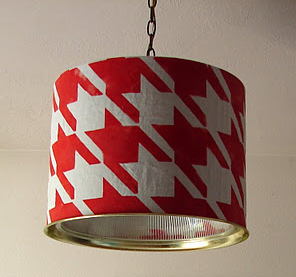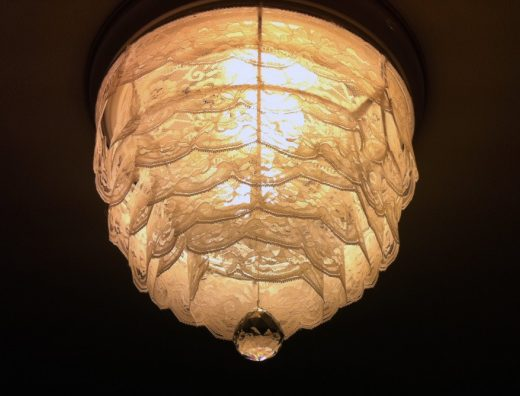Last Updated on January 8, 2016 by Kristi Linauer

This blue twig chandelier from The V Spot is so creative! The freeform shape is really great for this outdoor setting, and the color is fantastic.

Via The V Spot.

``` ```
``` On May 7, 2011 • By Kristi Linauer 2 You Might Also Like...Horchow-Inspired Natural Cane Lampshade July 18, 2012Outdated Lighting Turned Drum Pendant Light September 26, 2011DIY Lace Chandelier February 8, 2012 Previous Post Painted & Wallpapered Desk Next Post Welcome to Addicts (not so) Anonymous No. 4!! 2 Comments Leave a Comment Name * Email * Website Δdocument.getElementById( "ak_js_1" ).setAttribute( "value", ( new Date() ).getTime() ); This site uses Akismet to reduce spam. Learn how your comment data is processed. Reply To This Comment ↓ Kim Perdew May 7, 2011 at 11:52 pm HOW COOL…. HMMMM Makes me think I need one… :o) Love the color too. Reply To This Comment ↓ Vivienne @ The V Spot May 11, 2011 at 1:32 am Oh wow! Thanks for the shout out, Kristi! ```
``` ```
``` ```
``` ```
``` Well, hello there! I’m Kristi…I'm a DIY fanatic remodeling my way through our 1948 fixer upper.More About Kristi Addicted 2 Decorating is a participant in the Amazon Services LLC Associates Program, an affiliate advertising program designed to provide a means for sites to earn advertising fees by advertising and linking to Amazon.com. ```
``` ```
``` addicted2decorating I was scrolling through Instagram and Matt heard tI finished the water closet area of our master batI just snapped this picture because the sunlight wThere have been a couple of changes in here. FirstI made a flush mount glass bubble chandelier to goLoad More... Follow on Instagram Facebook Twitter Instagram Pinterest Youtube Copyright 2007-2022 - Kristi Linauer, LLC. All Rights Reserved. Addicted 2 Decorating is a participant in the Amazon Services LLC Associates Program, an affiliate advertising program designed to provide a means for sites to earn advertising fees by advertising and linking to Amazon.com. Privacy Policy Terms and Conditions ```
``` ```
``` !function(){"use strict";var e=function(e,t){return null==e||e!=e?t:e},t=function(e){var t=e.offsetHeight,n=e.offsetWidth,i=e.getBoundingClientRect(),o=document.body,r=document.documentElement,a=window.pageYOffset||r.scrollTop||o.scrollTop,l=window.pageXOffset||r.scrollLeft||o.scrollLeft,s=r.clientTop||o.clientTop||0,c=r.clientLeft||o.clientLeft||0,u=Math.round(i.top+a-s),d=Math.round(i.left+l-c);return{top:u,left:d,bottom:u+t,right:d+n,width:n,height:t}},n=function(e,t){return n=Object.setPrototypeOf||{__proto__:[]}instanceof Array&&function(e,t){e.__proto__=t}||function(e,t){for(var n in t)Object.prototype.hasOwnProperty.call(t,n)&&(e[n]=t[n])},n(e,t)};function i(e,t){if("function"!=typeof t&&null!==t)throw new TypeError("Class extends value "+String(t)+" is not a constructor or null");function i(){this.constructor=e}n(e,t),e.prototype=null===t?Object.create(t):(i.prototype=t.prototype,new i)}var o=function(){return o=Object.assign||function(e){for(var t,n=1,i=arguments.length;n<i;n++)for(var o in t=arguments[n])Object.prototype.hasOwnProperty.call(t,o)&&(e[o]=t[o]);return e},o.apply(this,arguments)};function r(e,t,n,i){var o,r=arguments.length,a=r<3?t:null===i?i=Object.getOwnPropertyDescriptor(t,n):i;if("object"==typeof Reflect&&"function"==typeof Reflect.decorate)a=Reflect.decorate(e,t,n,i);else for(var l=e.length-1;l>=0;l--)(o=e[l])&&(a=(r<3?o(a):r>3?o(t,n,a):o(t,n))||a);return r>3&&a&&Object.defineProperty(t,n,a),a}function a(e,t){if("object"==typeof Reflect&&"function"==typeof Reflect.metadata)return Reflect.metadata(e,t)}function l(e){var t="function"==typeof Symbol&&Symbol.iterator,n=t&&e[t],i=0;if(n)return n.call(e);if(e&&"number"==typeof e.length)return{next:function(){return e&&i>=e.length&&(e=void 0),{value:e&&e[i++],done:!e}}};throw new TypeError(t?"Object is not iterable.":"Symbol.iterator is not defined.")}function s(e,t){var n="function"==typeof Symbol&&e[Symbol.iterator];if(!n)return e;var i,o,r=n.call(e),a=[];try{for(;(void 0===t||t-- >0)&&!(i=r.next()).done;)a.push(i.value)}catch(e){o={error:e}}finally{try{i&&!i.done&&(n=r.return)&&n.call(r)}finally{if(o)throw o.error}}return a}function c(e,t,n){if(n||2===arguments.length)for(var i,o=0,r=t.length;o<r;o++)!i&&o in t||(i||(i=Array.prototype.slice.call(t,0,o)),i[o]=t[o]);return e.concat(i||Array.prototype.slice.call(t))}var u,d=function(e){sessionStorage.removeItem("adthrive_".concat(e.toLowerCase()))},p=function(e,t,n){void 0===n&&(n=!1);try{var i=sessionStorage.getItem("adthrive_".concat(e.toLowerCase()));if(i){var o=JSON.parse(i),r=void 0!==t&&Date.now()-o.created>=t;if(o&&!r)return n&&h(e,o.value),o.value}}catch(e){}return null},h=function(e,t){try{var n={value:t,created:Date.now()};sessionStorage.setItem("adthrive_".concat(e.toLowerCase()),JSON.stringify(n))}catch(e){}},y=function(e,t,n,i,r){var a;void 0===i&&(i=!0);var l="abgroup",s=p(l,n,i);if(null!==s){var c=s[e];if(void 0!==c)return c}var u=t(),d=o(o({},s),((a={})[e]=u,a));return h(l,d),r&&r(),u},f=function(e){return void 0===e&&(e=navigator.userAgent),/Windows NT|Macintosh/i.test(e)},m=function(){try{return Math.round(window.performance.now())}catch(e){return 0}},g=function(e){try{return{valid:!0,elements:document.querySelectorAll(e)}}catch(e){return o({valid:!1},e)}},_=function(e){return""===e?{valid:!0}:g(e)},v=function(){function e(){}return e.prototype.info=function(e,t){for(var n=[],i=2;i<arguments.length;i++)n[i-2]=arguments[i];this.call.apply(this,c([console.info,e,t],s(n),!1))},e.prototype.warn=function(e,t){for(var n=[],i=2;i<arguments.length;i++)n[i-2]=arguments[i];this.call.apply(this,c([console.warn,e,t],s(n),!1))},e.prototype.error=function(e,t){for(var n=[],i=2;i<arguments.length;i++)n[i-2]=arguments[i];this.call.apply(this,c([console.error,e,t],s(n),!1)),this.sendErrorLogToCommandQueue.apply(this,c([e,t],s(n),!1))},e.prototype.event=function(e,t){for(var n=[],i=2;i<arguments.length;i++)n[i-2]=arguments[i];this.info(e,t)},e.prototype.sendErrorLogToCommandQueue=function(e,t){for(var n=[],i=2;i<arguments.length;i++)n[i-2]=arguments[i];window.adthrive=window.adthrive||{},window.adthrive.cmd=window.adthrive.cmd||[],window.adthrive.cmd.push(function(){void 0!==window.adthrive.logError&&"function"==typeof window.adthrive.logError&&window.adthrive.logError(e,t,n)}.bind(e,t,n))},e.prototype.call=function(e,t,n){for(var i=[],o=3;o<arguments.length;o++)i[o-3]=arguments[o];var r=["%c".concat(t,"::").concat(n," ")],a=["color: #999; font-weight: bold;"];i.length>0&&"string"==typeof i&&r.push(i.shift()),a.push.apply(a,c([],s(i),!1));try{Function.prototype.apply.call(e,console,c([r.join("")],s(a),!1))}catch(e){return void console.error(e)}},e}(),b=new v,S=function(e,t){if(!e)return!1;var n=!!e.enabled,i=null==e.dateStart||Date.now()>=e.dateStart,o=null==e.dateEnd||Date.now()<=e.dateEnd,r=null===e.selector||""!==e.selector&&!!document.querySelector(e.selector),a="mobile"===e.platform&&"mobile"===t,l="desktop"===e.platform&&"desktop"===t,s=null===e.platform||"all"===e.platform||a||l,c="bernoulliTrial"===e.experimentType?P(e.variants):E(e.variants);return n&&i&&o&&r&&s&&c},P=function(e){return 1===e.length},E=function(e){var t=0;return e.forEach((function(e){e.weight&&(t+=e.weight)})),e.length>0&&e.every((function(e){var t=e.value,n=e.weight;return!(!t||!n)}))&&100===t},A=["siteId","siteName","adOptions","breakpoints","adUnits"],w=function(){function e(){this._clsGlobalData=window.adthriveCLS}return Object.defineProperty(e.prototype,"enabled",{get:function(){return!!this._clsGlobalData&&!!this._clsGlobalData.siteAds&&function(e,t){if(void 0===t&&(t=A),!e)return!1;for(var n=0;n<t.length;n++)if(!e[t[n]])return!1;return!0}(this._clsGlobalData.siteAds)},enumerable:!1,configurable:!0}),Object.defineProperty(e.prototype,"siteAds",{get:function(){return this._clsGlobalData.siteAds},set:function(e){this._clsGlobalData.siteAds=e},enumerable:!1,configurable:!0}),Object.defineProperty(e.prototype,"disableAds",{get:function(){return this._clsGlobalData.disableAds},set:function(e){this._clsGlobalData.disableAds=e},enumerable:!1,configurable:!0}),Object.defineProperty(e.prototype,"enabledLocations",{get:function(){return this._clsGlobalData.enabledLocations},set:function(e){this._clsGlobalData.enabledLocations=e},enumerable:!1,configurable:!0}),Object.defineProperty(e.prototype,"injectedFromPlugin",{get:function(){return this._clsGlobalData.injectedFromPlugin},set:function(e){this._clsGlobalData.injectedFromPlugin=e},enumerable:!1,configurable:!0}),Object.defineProperty(e.prototype,"injectedFromSiteAds",{get:function(){return this._clsGlobalData.injectedFromSiteAds},set:function(e){this._clsGlobalData.injectedFromSiteAds=e},enumerable:!1,configurable:!0}),e.prototype.overwriteInjectedSlots=function(e){this._clsGlobalData.injectedSlots=e},e.prototype.setInjectedSlots=function(e){this._clsGlobalData.injectedSlots=this._clsGlobalData.injectedSlots||[],this._clsGlobalData.injectedSlots.push(e)},Object.defineProperty(e.prototype,"injectedSlots",{get:function(){return this._clsGlobalData.injectedSlots},enumerable:!1,configurable:!0}),e.prototype.setInjectedVideoSlots=function(e){this._clsGlobalData.injectedVideoSlots=this._clsGlobalData.injectedVideoSlots||[],this._clsGlobalData.injectedVideoSlots.push(e)},Object.defineProperty(e.prototype,"injectedVideoSlots",{get:function(){return this._clsGlobalData.injectedVideoSlots},enumerable:!1,configurable:!0}),e.prototype.setInjectedScripts=function(e){this._clsGlobalData.injectedScripts=this._clsGlobalData.injectedScripts||[],this._clsGlobalData.injectedScripts.push(e)},Object.defineProperty(e.prototype,"getInjectedScripts",{get:function(){return this._clsGlobalData.injectedScripts},enumerable:!1,configurable:!0}),e.prototype.setExperiment=function(e,t,n){void 0===n&&(n=!1),this._clsGlobalData.experiments=this._clsGlobalData.experiments||{},this._clsGlobalData.siteExperiments=this._clsGlobalData.siteExperiments||{},(n?this._clsGlobalData.siteExperiments:this._clsGlobalData.experiments)[e]=t},e.prototype.getExperiment=function(e,t){void 0===t&&(t=!1);var n=t?this._clsGlobalData.siteExperiments:this._clsGlobalData.experiments;return n&&n[e]},e.prototype.setWeightedChoiceExperiment=function(e,t,n){void 0===n&&(n=!1),this._clsGlobalData.experimentsWeightedChoice=this._clsGlobalData.experimentsWeightedChoice||{},this._clsGlobalData.siteExperimentsWeightedChoice=this._clsGlobalData.siteExperimentsWeightedChoice||{},(n?this._clsGlobalData.siteExperimentsWeightedChoice:this._clsGlobalData.experimentsWeightedChoice)[e]=t},e.prototype.getWeightedChoiceExperiment=function(e,t){var n,i;void 0===t&&(t=!1);var o=t?null===(n=this._clsGlobalData)||void 0===n?void 0:n.siteExperimentsWeightedChoice:null===(i=this._clsGlobalData)||void 0===i?void 0:i.experimentsWeightedChoice;return o&&o[e]},Object.defineProperty(e.prototype,"branch",{get:function(){return this._clsGlobalData.branch},enumerable:!1,configurable:!0}),Object.defineProperty(e.prototype,"bucket",{get:function(){return this._clsGlobalData.bucket},enumerable:!1,configurable:!0}),Object.defineProperty(e.prototype,"videoDisabledFromPlugin",{get:function(){return this._clsGlobalData.videoDisabledFromPlugin},set:function(e){this._clsGlobalData.videoDisabledFromPlugin=e},enumerable:!1,configurable:!0}),Object.defineProperty(e.prototype,"targetDensityLog",{get:function(){return this._clsGlobalData.targetDensityLog},set:function(e){this._clsGlobalData.targetDensityLog=e},enumerable:!1,configurable:!0}),e}(),O=function(){function e(){}return e.getScrollTop=function(){return(window.pageYOffset||document.documentElement.scrollTop)-(document.documentElement.clientTop||0)},e.shufflePlaylist=function(e){for(var t,n,i=e.length;0!==i;)n=Math.floor(Math.random()*e.length),t=e[i-=1],e[i]=e[n],e[n]=t;return e},e.isMobileLandscape=function(){return window.matchMedia("(orientation: landscape) and (max-height: 480px)").matches},e.playerViewable=function(e){var t=e.getBoundingClientRect();return this.isMobileLandscape()?window.innerHeight>t.top+t.height/2&&t.top+t.height/2>0:window.innerHeight>t.top+t.height/2},e.playerVisible=function(e){var t=e.getBoundingClientRect();return window.innerHeight>t.top},e.createQueryString=function(e){return Object.keys(e).map((function(t){return"".concat(t,"=").concat(e[t])})).join("&")},e.createEncodedQueryString=function(e){return Object.keys(e).map((function(t){return"".concat(t,"=").concat(encodeURIComponent(e[t]))})).join("&")},e.setMobileLocation=function(e){var t,n;return"top-left"===(e=e||"bottom-right")?e="adthrive-collapse-top-left":"top-right"===e?e="adthrive-collapse-top-right":"bottom-left"===e?e="adthrive-collapse-bottom-left":"bottom-right"===e?e="adthrive-collapse-bottom-right":"top-center"===e&&(t=navigator.userAgent,n=/Tablet|iPad|Playbook|Nook|webOS|Kindle|Android (?!.*Mobile).*Safari/i.test(t),e=/Mobi|iP(hone|od)|Opera Mini/i.test(t)&&!n?"adthrive-collapse-top-center":"adthrive-collapse-bottom-right"),e},e}(),D=function(t){this._clsOptions=t,this.removeVideoTitleWrapper=e(this._clsOptions.siteAds.adOptions.removeVideoTitleWrapper,!1);var n=this._clsOptions.siteAds.videoPlayers;this.footerSelector=e(n&&n.footerSelector,""),this.players=e(n&&n.players.map((function(e){return e.mobileLocation=O.setMobileLocation(e.mobileLocation),e})),[]),this.contextualSettings=n&&n.contextual},C=function(e){this.mobileStickyPlayerOnPage=!1,this.playlistPlayerAdded=!1,this.contextualPlayerAdded=!1,this.sekindoPlayerAdded=!1,this.footerSelector="",this.removeVideoTitleWrapper=!1,this.videoAdOptions=new D(e),this.players=this.videoAdOptions.players,this.contextualSettings=this.videoAdOptions.contextualSettings,this.removeVideoTitleWrapper=this.videoAdOptions.removeVideoTitleWrapper,this.footerSelector=this.videoAdOptions.footerSelector},M=function(){},x=function(e){function t(t){var n=e.call(this)||this;return n._probability=t,n}return i(t,e),t.prototype.get=function(){if(this._probability<0||this._probability>1)throw new Error("Invalid probability");return Math.random()<this._probability},t}(M),I=function(){function e(){this._clsOptions=new w,this.shouldUseCoreOverride=!1}return e.prototype.setExperimentKey=function(e){void 0===e&&(e=!1),this._clsOptions.setExperiment(this.key,this.result,e)},e}(),k=function(e){function t(){var t=e.call(this)||this;return t._result=!1,t._choices=[{choice:!0},{choice:!1}],t.key="smhd100",t._result=t.run(),t.setExperimentKey(),t}return i(t,e),Object.defineProperty(t.prototype,"result",{get:function(){return this._result},enumerable:!1,configurable:!0}),t.prototype.run=function(){return new x(.1).get()},t}(I),j=[[728,90],[300,250],[300,600],[320,50],[970,250],[160,600],[300,1050],[336,280],[970,90],[300,50],[320,100],[468,60],[250,250],[120,240],[1,1],[300,300],[552,334],[300,420],[728,250],[320,300],[300,390]],L=new Map([["Footer",1],["Header",2],["Sidebar",3],["Content",4],["Recipe",5],["Sidebar_sticky",6],["Below Post",7]]),R=function(e,t){var n=function(e){var t=e.clientWidth;if(getComputedStyle){var n=getComputedStyle(e,null);t-=parseFloat(n.paddingLeft||"0")+parseFloat(n.paddingRight||"0")}return t}(t),i=e.sticky&&"Sidebar"===e.location;return e.sizes.filter((function(t){var o=!e.autosize||(t<=n||t<=320),r=!i||t<=window.innerHeight-100;return o&&r}))},T=function(e){return j.filter((function(t){var n=s(t,2),i=n,o=n;return e.some((function(e){var t=s(e,2),n=t,r=t;return i===n&&o===r}))}))},G=function(e,t,n,i){var o=s(t,2),r=o,a=o;return!("Footer"===e&&"phone"===n&&320===r&&100===a||"Header"===e&&a>100&&i.result)},H=function(e){var t,n,i=f()?"desktop":"mobile";this.siteExperiments=null===(n=null===(t=e.siteAds)||void 0===t?void 0:t.siteExperiments)||void 0===n?void 0:n.filter((function(e){return S(e,i)}))},V=function(e){this.clsOptions=e,this.enabledLocations=["Below_Post","Content","Recipe","Sidebar"],this.site=new H(e)};!function(e){e.Desktop="desktop",e.Mobile="mobile"}(u||(u={}));var W,F,N,z,B,q,U,K,J=function(e){function t(t,n){void 0===t&&(t=[]);var i=e.call(this)||this;return i._choices=t,i._default=n,i}return i(t,e),t.fromArray=function(e,n){return new t(e.map((function(e){var t=s(e,2);return{choice:t,weight:t}})),n)},t.prototype.addChoice=function(e,t){this._choices.push({choice:e,weight:t})},t.prototype.get=function(){var e,t,n,i,o=(n=0,i=100,Math.random()*(i-n)+n),r=0;try{for(var a=l(this._choices),s=a.next();!s.done;s=a.next()){var c=s.value,u=c.choice;if((r+=c.weight)>=o)return u}}catch(t){e={error:t}}finally{try{s&&!s.done&&(t=a.return)&&t.call(a)}finally{if(e)throw e.error}}return this._default},Object.defineProperty(t.prototype,"totalWeight",{get:function(){return this._choices.reduce((function(e,t){return e+t.weight}),0)},enumerable:!1,configurable:!0}),t}(M);!function(e){e.Prebid="prebid",e.GAM="gam",e.Amazon="amazon",e.WebVitals="webvitals",e.Marmalade="marmalade",e.Floors="floors",e.CMP="cmp"}(W||(W={})),function(e){e.prebidLoad="prebidLoad",e.amazonLoad="amazonLoad",e.gptLoad="gptLoad",e.rubiconFloors="rubiconFloors"}(F||(F={})),function(e){e.Video_Collapse_Autoplay_SoundOff="Video_Collapse_Autoplay_SoundOff",e.Video_Individual_Autoplay_SOff="Video_Individual_Autoplay_SOff",e.Video_Coll_SOff_Smartphone="Video_Coll_SOff_Smartphone",e.Video_In_Post_ClicktoPlay_SoundOn="Video_In-Post_ClicktoPlay_SoundOn"}(N||(N={})),function(e){e.StickySekindo="stickySekindo",e.StaticSekindo="staticSekindo",e.None="none"}(z||(z={})),function(e){e.WEIGHTEDCHOICE="weightedChoice",e.BERNOULLITRIAL="bernoulliTrial"}(B||(B={})),function(e){e.INTEGER="integer",e.FLOAT="float",e.BOOLEAN="boolean",e.STRING="string"}(q||(q={})),function(e){e.DESKTOP="desktop",e.MOBILE="mobile",e.ALL="all"}(U||(U={})),function(e){e.AdDensity="addensity",e.FooterCloseButton="footerclose",e.Interstitial="interstitial",e.RemoveVideoTitleWrapper="removevideotitlewrapper",e.StickyOutstream="stickyoutstream",e.StickyOutstreamOnStickyPlayer="sospp",e.VideoAdvancePlaylistRelatedPlayer="videoadvanceplaylistrp",e.MobileStickyPlayerPosition="mspp"}(K||(K={}));var Q,Y=function(){function e(){}return Object.defineProperty(e.prototype,"enabled",{get:function(){return void 0!==this.experimentConfig},enumerable:!1,configurable:!0}),e}(),X=function(){function e(e){var t;this.siteExperiments=[],this._clsOptions=e;var n=this._clsOptions.siteAds.siteExperiments,i=f()?"desktop":"mobile";this.siteExperiments=null!==(t=null==n?void 0:n.filter((function(e){return S(e,i)})))&&void 0!==t?t:[]}return e.prototype.getSiteExperimentByKey=function(e){return this.siteExperiments.filter((function(t){return t.key.toLowerCase()===e.toLowerCase()}))},e}(),Z=function(e,t,n,i,o,r){var a=Math.round(r-o),l=[],u=[];l.push("(",n.map((function(){return"%o"})).join(", "),")"),u.push.apply(u,c([],s(n),!1)),void 0!==i&&(l.push(" => %o"),u.push(i)),l.push(" %c(".concat(a,"ms)")),u.push("color: #999;")},\$=function(e,t,n){var i=void 0!==n.get?n.get:n.value;return function(){for(var e=[],t=0;t<arguments.length;t++)e[t]=arguments[t];try{var n=m(),o=i.apply(this,e);if(o instanceof Promise)return o.then((function(t){var i=m();return Z(0,0,e,t,n,i),Promise.resolve(t)})).catch((function(e){throw e.logged||(e.logged=!0),e}));var r=m();return Z(0,0,e,o,n,r),o}catch(e){throw e.logged||(e.logged=!0),e}}},ee=function(e){function t(t){var n=e.call(this)||this;n._choices=[],n._result=null,n.key=K.AdDensity;var i=new X(t);return n.experimentConfig=i.getSiteExperimentByKey(n.key),n.experimentConfig&&(n._choices=n.experimentConfig.variants,n._result=n.run(),null!==n._result&&t.setWeightedChoiceExperiment(n.key,n._result,!0)),n}var n;return i(t,e),Object.defineProperty(t.prototype,"result",{get:function(){return this._result},enumerable:!1,configurable:!0}),t.prototype.run=function(){var e;this.experimentConfig;var t=this._choices.map((function(e){return{choice:e.value/100,weight:e.weight}}));return null!==(e=new J(t).get())&&void 0!==e?e:null},r([function(e,t,n){var i=n.value;i&&(n.value=function(){for(var e,t=this,n=[],o=0;o<arguments.length;o++)n[o]=arguments[o];var r=this.sessionStoragePrefix?"".concat(this.sessionStoragePrefix,"-").concat(this.key):this.key;return!1===(null===(e=p("branch"))||void 0===e?void 0:e.enabled)&&d(r),y(r,(function(){return i.apply(t,n)}),18e5)})},a("design:type",Function),a("design:paramtypes",[]),a("design:returntype",void 0)],t.prototype,"run",null),t=r([(void 0===n&&(n=!1),function(e){var t,i,r=Object.getOwnPropertyNames(e.prototype).filter((function(e){return n||0!==e.indexOf("_")})).map((function(t){return[t,Object.getOwnPropertyDescriptor(e.prototype,t)]}));try{for(var a=l(r),c=a.next();!c.done;c=a.next()){var u=s(c.value,2),d=u,p=u;void 0!==p&&"function"==typeof p.value?e.prototype[d]=\$(0,0,p):void 0!==p&&void 0!==p.get&&"function"==typeof p.get&&Object.defineProperty(e.prototype,d,o(o({},p),{get:\$(0,0,p)}))}}catch(e){t={error:e}}finally{try{c&&!c.done&&(i=a.return)&&i.call(a)}finally{if(t)throw t.error}}}),a("design:paramtypes",[w])],t)}(Y),te="250px",ne=function(){function n(e,t){this._clsOptions=e,this._adInjectionMap=t,this._totalContentInserted=0,this._recipeCount=0,this._mainContentHeight=0,this._mainContentDiv=null,this._totalAvailableElements=[],this._minDivHeight=250,this._densityDevice=u.Desktop,this._pubLog={onePerViewport:!1,targetDensity:0,targetDensityUnits:0,combinedMax:0},this._densityMax=.99,this._smallerIncrementAttempts=0,this._absoluteMinimumSpacingByDevice=250,this._usedAbsoluteMinimum=!1,this._infPageEndOffset=0,this.locationMaxLazySequence=new Map([["Recipe",5]]),this.locationToMinHeight={Below_Post:te,Content:te,Recipe:te,Sidebar:te};var n=this._clsOptions.siteAds.breakpoints,i=n.tablet,o=n.desktop;this._device=function(e,t){var n=window.innerWidth;return n>=t?"desktop":n>=e?"tablet":"phone"}(i,o),this._config=new V(e),this._clsOptions.enabledLocations=this._config.enabledLocations,this._clsAdDensitySiteExperiment=this._clsOptions.siteAds.siteExperiments?new ee(this._clsOptions):null}return n.prototype.start=function(){var t,n,i,o=this;try{!function(e){var t=document.body,n="adthrive-device-".concat(e);if(!t.classList.contains(n))try{t.classList.add(n)}catch(e){document.createElement("_")}}(this._device);var r=(t=this._device,n=this._clsOptions.siteAds.adUnits,i=new k,n.filter((function(e){return void 0!==e.dynamic&&e.dynamic.enabled})).map((function(n){var o=n.location.replace(/\s+/g,"_");return{auctionPriority:L.get(o)||8,location:o,sequence:e(n.sequence,1),sizes:T(n.adSizes).filter((function(e){return G(n.location,e,t,i)})),devices:n.devices,pageSelector:e(n.dynamic.pageSelector,"").trim(),elementSelector:e(n.dynamic.elementSelector,"").trim(),position:e(n.dynamic.position,"beforebegin"),max:Math.floor(e(n.dynamic.max,0)),spacing:e(n.dynamic.spacing,0),skip:Math.floor(e(n.dynamic.skip,0)),every:Math.max(Math.floor(e(n.dynamic.every,1)),1),classNames:n.dynamic.classNames||[],sticky:"Footer"===n.location||n.sticky,stickyOverlapSelector:e(n.stickyOverlapSelector,"").trim(),autosize:n.autosize,special:e(n.targeting,[]).filter((function(e){return"special"===e.key})).reduce((function(e,t){return e.concat.apply(e,c([],s(t.value),!1))}),[]),lazy:e(n.dynamic.lazy,!1),lazyMax:e(n.dynamic.lazyMax,2),lazyMaxDefaulted:0!==n.dynamic.lazyMax&&!n.dynamic.lazyMax}}))).filter((function(e){return o._locationEnabled(e)})).filter((function(e){return function(e,t){return e.devices.includes(t)}(e,o._device)})).filter((function(e){return 0===(t=e).pageSelector.length||null!==document.querySelector(t.pageSelector);var t}));this.inject(r).forEach((function(e){return o._clsOptions.setInjectedSlots(e)}))}catch(e){}},n.prototype.inject=function(t,n){void 0===n&&(n=document),this._densityDevice="desktop"===this._device?u.Desktop:u.Mobile,this._overrideDefaultAdDensitySettingsWithSiteExperiment();var i=this._clsOptions.siteAds,o=e(i.adDensityEnabled,!0),r=i.adDensityLayout&&o,a=t.filter((function(e){return r?"Content"!==e.location:e})),l=t.filter((function(e){return r?"Content"===e.location:null}));return c(c([],s(a.length?this._injectNonDensitySlots(a,n):[]),!1),s(l.length?this._injectDensitySlots(l,n):[]),!1)},n.prototype._injectNonDensitySlots=function(e,n){var i,o,r=this;void 0===n&&(n=document);var a=[],u=[];try{for(var d=l(e),p=d.next();!p.done;p=d.next()){var h=p.value,y=0,f=0,m=0;h.spacing>0&&(y=window.innerHeight*h.spacing,f=y);var g=this._repeatDynamicAds(h),_=this.getElements(h.elementSelector,n);h.skip;for(var v=function(e){if(m+1>g.length)return"break";var i=_[e];if(y>0){var o=t(i).bottom;if(o<=f)return"continue";f=o+y}var r=g[m],l="".concat(r.location,"_").concat(r.sequence);a.some((function(e){return e.name===l}))&&(m+=1);var d=b.getDynamicElementId(r),p="adthrive-".concat(h.location.replace("_","-").toLowerCase()),v=c([p,"".concat(p,"-").concat(h.sequence)],s(h.classNames),!1),S=b.addAd(i,d,h.position,v);if(S){var P=R(r,S);if(P.length){var E={clsDynamicAd:h,dynamicAd:r,element:S,sizes:P,name:l,infinite:n!==document};a.push(E),u.push({location:r.location,element:S}),"Recipe"===h.location&&++b._recipeCount,m+=1}i=S}},b=this,S=h.skip;S<_.length;S+=h.every){if("break"===v(S))break}}}catch(e){i={error:e}}finally{try{p&&!p.done&&(o=d.return)&&o.call(d)}finally{if(i)throw i.error}}return u.forEach((function(e){var t=e.location;e.element.style.minHeight=r.locationToMinHeight[t]})),a},n.prototype._injectDensitySlots=function(e,t){void 0===t&&(t=document);try{this._calculateMainContentHeightAndAllElements(e,t)}catch(e){return[]}var n=this._getDensitySettings(e,t),i=n.onePerViewport,o=n.targetAll,r=n.targetDensityUnits,a=n.combinedMax,l=n.numberOfUnits;return this._absoluteMinimumSpacingByDevice=i?window.innerHeight:this._absoluteMinimumSpacingByDevice,l?(this._adInjectionMap.filterUsed(),this._findElementsForAds(l,i,o,a,r,t),this._insertAds()):[]},n.prototype._overrideDefaultAdDensitySettingsWithSiteExperiment=function(){if(this._clsAdDensitySiteExperiment){var e=this._clsAdDensitySiteExperiment,t=e.enabled,n=e.result;t&&"number"==typeof n&&(this._clsOptions.siteAds.adDensityEnabled=!0,this._clsOptions.siteAds.adDensityLayout[this._densityDevice].adDensity=n)}},n.prototype._getDensitySettings=function(e,t){void 0===t&&(t=document);var n=this._clsOptions.siteAds.adDensityLayout,i=this._determineOverrides(n.pageOverrides),o=i.length?i:n[this._densityDevice],r=o.adDensity,a=o.onePerViewport,l=this._shouldTargetAllEligible(r),u=this._getTargetDensityUnits(r,l),d=this._getCombinedMax(e,t),p=Math.min.apply(Math,c([],s(c([this._totalAvailableElements.length,u],s(d>0?[d]:[]),!1)),!1));return this._pubLog={onePerViewport:a,targetDensity:r,targetDensityUnits:u,combinedMax:d},{onePerViewport:a,targetAll:l,targetDensityUnits:u,combinedMax:d,numberOfUnits:p}},n.prototype._determineOverrides=function(e){var t=this;return e.filter((function(e){var t=_(e.pageSelector);return""===e.pageSelector||t.elements&&t.elements.length})).map((function(e){return e[t._densityDevice]}))},n.prototype._shouldTargetAllEligible=function(e){return e===this._densityMax},n.prototype._getTargetDensityUnits=function(e,t){return t?this._totalAvailableElements.length:Math.floor(e*this._mainContentHeight/(1-e)/this._minDivHeight)-this._recipeCount},n.prototype._getCombinedMax=function(t,n){return void 0===n&&(n=document),e(t.filter((function(e){var t;try{t=n.querySelector(e.elementSelector)}catch(e){}return t})).map((function(e){return Number(e.max)+Number(e.lazyMaxDefaulted?0:e.lazyMax)})).sort((function(e,t){return t-e})),0)},n.prototype._elementLargerThanMainContent=function(e){return e.offsetHeight>=this._mainContentHeight&&this._totalAvailableElements.length>1},n.prototype._elementDisplayNone=function(e){var t=window.getComputedStyle(e,null).display;return t&&"none"===t||"none"===e.style.display},n.prototype._isBelowMaxes=function(e,t){return this._adInjectionMap.map.length<e&&this._adInjectionMap.map.length<t},n.prototype._findElementsForAds=function(e,t,n,i,o,r){var a=this;void 0===r&&(r=document),this._clsOptions.targetDensityLog={onePerViewport:t,combinedMax:i,targetDensityUnits:o,targetDensityPercentage:this._pubLog.targetDensity,mainContentHeight:this._mainContentHeight,recipeCount:this._recipeCount,numberOfEls:this._totalAvailableElements.length};var s=function(e){var t,c;try{for(var u=l(a._totalAvailableElements),d=u.next();!d.done;d=u.next()){var p=d.value,h=p.dynamicAd,y=p.element;if(a._logDensityInfo(y,h.elementSelector,e),!(!n&&a._elementLargerThanMainContent(y)||a._elementDisplayNone(y))){if(!a._isBelowMaxes(i,o))break;a._checkElementSpacing({dynamicAd:h,element:y,insertEvery:e,targetAll:n,target:r})}}}catch(e){t={error:e}}finally{try{d&&!d.done&&(c=u.return)&&c.call(u)}finally{if(t)throw t.error}}!a._usedAbsoluteMinimum&&a._smallerIncrementAttempts<5&&(++a._smallerIncrementAttempts,s(a._getSmallerIncrement(e)))},c=this._getInsertEvery(e,t,o);s(c)},n.prototype._getSmallerIncrement=function(e){var t=.6*e;return t<=this._absoluteMinimumSpacingByDevice&&(t=this._absoluteMinimumSpacingByDevice,this._usedAbsoluteMinimum=!0),t},n.prototype._insertAds=function(){var e=this,t=[];return this._adInjectionMap.filterUsed(),this._adInjectionMap.map.forEach((function(n,i){var o=n.el;n.coords;var r=n.dynamicAd,a=n.target,l=Number(r.sequence)+i,s=r.max,c=r.lazy&&l>s;r.sequence=l,r.lazy=c;var u=e._addContentAd(o,r,a);u&&(r.used=!0,t.push(u))})),t},n.prototype._getInsertEvery=function(e,t,n){var i=this._absoluteMinimumSpacingByDevice;return this._moreAvailableElementsThanUnitsToInject(n,e)?(this._usedAbsoluteMinimum=!1,i=this._useWiderSpacing(n,e)):(this._usedAbsoluteMinimum=!0,i=this._useSmallestSpacing(t)),t&&window.innerHeight>i?window.innerHeight:i},n.prototype._useWiderSpacing=function(e,t){return this._mainContentHeight/Math.min(e,t)},n.prototype._useSmallestSpacing=function(e){return e&&window.innerHeight>this._absoluteMinimumSpacingByDevice?window.innerHeight:this._absoluteMinimumSpacingByDevice},n.prototype._moreAvailableElementsThanUnitsToInject=function(e,t){return this._totalAvailableElements.length>e||this._totalAvailableElements.length>t},n.prototype._logDensityInfo=function(e,t,n){var i=this._pubLog;i.onePerViewport,i.targetDensity;i.targetDensityUnits;i.combinedMax,this._totalAvailableElements.length},n.prototype._checkElementSpacing=function(e){var t=e.dynamicAd,n=e.element,i=e.insertEvery,r=e.targetAll,a=e.target,l=void 0===a?document:a;(this._isFirstAdInjected()||this._hasProperSpacing(n,t,r,i))&&(++this._totalContentInserted,this._markSpotForContentAd(n,o({},t),l))},n.prototype._isFirstAdInjected=function(){return!this._adInjectionMap.map.length},n.prototype._markSpotForContentAd=function(e,t,n){void 0===n&&(n=document);var i="beforebegin"===t.position||"afterbegin"===t.position;this._adInjectionMap.add(e,this._getElementCoords(e,i),t,n),this._adInjectionMap.sort()},n.prototype._hasProperSpacing=function(e,t,n,i){var o="beforebegin"===t.position||"afterbegin"===t.position,r="beforeend"===t.position||"afterbegin"===t.position,a=n||this._isElementFarEnoughFromOtherAdElements(e,i,o),l=r||this._isElementNotInRow(e,o),s=-1===e.id.indexOf("AdThrive_".concat("Below_Post"));return a&&l&&s},n.prototype._isElementFarEnoughFromOtherAdElements=function(e,t,n){for(var i=this._getElementCoords(e,n),o=!1,r=0;r<this._adInjectionMap.map.length;r++){var a=this._adInjectionMap.map[r].coords,l=this._adInjectionMap.map[r+1]&&this._adInjectionMap.map[r+1].coords;if(o=i-t>a&&(!l||i+t<l))break}return o},n.prototype._isElementNotInRow=function(e,t){var n=e.previousElementSibling,i=e.nextElementSibling,o=t?!n&&i||n&&e.tagName!==n.tagName?i:n:i;return!(!o||0!==e.getBoundingClientRect().height)||(!o||e.getBoundingClientRect().top!==o.getBoundingClientRect().top)},n.prototype._calculateMainContentHeightAndAllElements=function(e,t){void 0===t&&(t=document);var n=this._setMainContentAndElements(e,t);this._mainContentDiv=n;var i=this._mainContentDiv.querySelector("div #comments, section .comments");this._mainContentHeight=i?this._mainContentDiv.offsetHeight-i.offsetHeight:this._mainContentDiv.offsetHeight},n.prototype._setMainContentAndElements=function(e,t){void 0===t&&(t=document);var n=this._getElementsFromDynamicAdEntry(e,t);if(0===n.length)throw Error("No Main Content Elements Found");return Array.from(n).reduce((function(e,t){return t.offsetHeight>e.offsetHeight?t:e}))||document.body},n.prototype._getElementsFromDynamicAdEntry=function(e,t){var n=this;void 0===t&&(t=document);var i=this._getLongestArticleTag(t),o=i?[i]:[],r=[];e.forEach((function(e){var i=Array.from(n.getElements(e.elementSelector,t)).slice(0,e.skip);n._getSelectors(e.elementSelector).forEach((function(a){for(var l=t.querySelectorAll(a),s=function(t){var a=l[t];if(n._adInjectionMap.map.some((function(e){return e.el.isEqualNode(a)})))return"continue";var s=a&&a.parentElement;s&&s!==document.body?o.push(s):o.push(a),-1===i.indexOf(a)&&r.push({dynamicAd:e,element:a})},c=0;c<l.length;c++)s(c)}))}));var a=this._getContainerDivTop(t);return this._totalAvailableElements=r.sort((function(e,t){return e.element.getBoundingClientRect().top-a-(t.element.getBoundingClientRect().top-a)})),o},n.prototype._getContainerDivTop=function(e){return void 0===e&&(e=document),(e===document?document.body:e).getBoundingClientRect().top},n.prototype._getLongestArticleTag=function(e){void 0===e&&(e=document);var t=e.querySelectorAll("article");if(0===t.length)return null;var n=Array.from(t).reduce((function(e,t){return t.offsetHeight>e.offsetHeight?t:e}));return n&&n.offsetHeight>1.5*window.innerHeight?n:null},n.prototype._getSelectors=function(e){return e.includes(",")?e.split(","):[e]},n.prototype._getElementCoords=function(e,t){void 0===t&&(t=!1);var n=e.getBoundingClientRect();return(t?n.top:n.bottom)+window.scrollY},n.prototype._addContentAd=function(e,t,n){void 0===n&&(n=document);var i=null,o="adthrive-".concat(t.location.replace("_","-").toLowerCase()),r="".concat(o,"-").concat(t.sequence),a=this.addAd(e,this.getDynamicElementId(t),t.position,c([o,r],s(t.classNames),!1));if(a){var l=R(t,a);if(l.length)a.style.minHeight=this.locationToMinHeight[t.location],i={clsDynamicAd:t,dynamicAd:t,element:a,sizes:l,name:"".concat(t.location,"_").concat(t.sequence),infinite:n!==document}}return i},n.prototype.getDynamicElementId=function(e){return"".concat("AdThrive","_").concat(e.location,"_").concat(e.sequence,"_").concat(this._device)},n.prototype.getElements=function(e,t){return void 0===t&&(t=document),t.querySelectorAll(e)},n.prototype.addAd=function(e,t,n,i){if(void 0===i&&(i=[]),!document.getElementById(t)){var o='<div id="'.concat(t,'" class="adthrive-ad ').concat(i.join(" "),'"></div>');e.insertAdjacentHTML(n,o)}return document.getElementById(t)},n.prototype._repeatDynamicAds=function(t){for(var n=[],i=t.lazy?e(this.locationMaxLazySequence.get(t.location),0):0,r=t.max,a=t.lazyMax,l=0===i&&t.lazy?r+a:Math.min(Math.max(i-t.sequence+1,0),r+a),s=Math.max(r,l),c=0;c<s;c++){var u=Number(t.sequence)+c,d=t.lazy&&c>=r;n.push(o(o({},t),{sequence:u,lazy:d}))}return n},n.prototype._locationEnabled=function(e){var t=this._clsOptions.enabledLocations.includes(e.location),n=this._clsOptions.disableAds&&this._clsOptions.disableAds.all||document.body.classList.contains("adthrive-disable-all"),i=!document.body.classList.contains("adthrive-disable-content")&&!this._clsOptions.disableAds.reasons.has("content_plugin");return t&&!n&&i},n}(),ie=function(e){function t(){var t=e.call(this)||this;return t.key="sekho",t._result=!1,t._choices=[{choice:!0},{choice:!1}],t._result=t.run(),t.setExperimentKey(),t}return i(t,e),Object.defineProperty(t.prototype,"result",{get:function(){return this._result},enumerable:!1,configurable:!0}),t.prototype.run=function(){return new x(.05).get()},t}(I),oe=function(e){function t(t,n){var i=e.call(this,t,(new ie).result,"ClsVideoInsertion")||this;return i._videoConfig=t,i._clsOptions=n,i._IN_POST_SELECTOR=".adthrive-video-player",i._WRAPPER_BAR_HEIGHT=36,i._playersAddedFromPlugin=[],i}return i(t,e),t.prototype.init=function(){this._initializePlayers()},t.prototype._wrapJWPlayerWithCLS=function(e,t,n){if(void 0===n&&(n=0),e.parentNode){var i=e.offsetWidth*(9/16),o=this._createGenericCLSWrapper(i,t,n);return e.parentNode.insertBefore(o,e),o.appendChild(e),o}return null},t.prototype._createSekindoCLSWrapper=function(e,t){var n=this._getTitleHeight(document.createElement("h4"));return this._createGenericCLSWrapper(e,t,n)},t.prototype._createGenericCLSWrapper=function(e,t,n){var i=document.createElement("div");return i.id="cls-video-container-".concat(t),i.className="adthrive",i.style.minHeight="".concat(e+n,"px"),i},t.prototype._getTitleHeight=function(e){e.innerText="Title",e.style.visibility="hidden",document.body.appendChild(e);var t=window.getComputedStyle(e),n=parseInt(t.height,10),i=parseInt(t.marginTop,10),o=parseInt(t.marginBottom,10);return document.body.removeChild(e),Math.min(n+o+i,50)},t.prototype._initializePlayers=function(){var e=document.querySelectorAll(this._IN_POST_SELECTOR);e.length&&this._initializeRelatedPlayers(e),this._shouldRunAutoplayPlayers()&&this._determineAutoplayPlayers()},t.prototype._createStationaryRelatedPlayer=function(e,t){var n="mobile"===this._device?[400,225]:[640,360],i=N.Video_In_Post_ClicktoPlay_SoundOn;if(t&&e.mediaId){var o=this._wrapJWPlayerWithCLS(t,e.mediaId);this._playersAddedFromPlugin.push(e.mediaId),o&&this._clsOptions.setInjectedVideoSlots({playerId:e.playerId,playerName:i,playerSize:n,element:o,type:"stationaryRelated"})}},t.prototype._createStickyRelatedPlayer=function(e,t){var n="mobile"===this._device?[400,225]:[640,360],i=N.Video_Individual_Autoplay_SOff;if(this._stickyRelatedOnPage=!0,this._videoConfig.mobileStickyPlayerOnPage="mobile"===this._device,t&&e.position&&e.mediaId){var o=document.createElement("div");t.insertAdjacentElement(e.position,o);var r=document.createElement("h3");r.style.margin="10px 0";var a=this._getTitleHeight(r),l=this._wrapJWPlayerWithCLS(o,e.mediaId,this._WRAPPER_BAR_HEIGHT+a);this._playersAddedFromPlugin.push(e.mediaId),l&&this._clsOptions.setInjectedVideoSlots({playlistId:e.playlistId,playerId:e.playerId,playerSize:n,playerName:i,element:o,type:"stickyRelated"})}},t.prototype._createSekindoPlayer=function(e,t){var n=e.playlistId;o(o({},e),{classNames:[]});var i="mobile"===this._device?{width:340,height:260}:{width:320,height:250},r=this._createSekindoCLSWrapper(i.height,n);t.insertAdjacentElement(e.position,r),this._playersAddedFromPlugin.push(n),r&&this._clsOptions.setInjectedVideoSlots({playlistId:n,playerId:e.playerId,playerSize:[i.width,i.height],element:r,type:"sekindo"})},t.prototype._createPlaylistPlayer=function(e,t){var n=e.playlistId,i="mobile"===this._device?N.Video_Coll_SOff_Smartphone:N.Video_Collapse_Autoplay_SoundOff,o="mobile"===this._device?[400,225]:[640,360];this._videoConfig.mobileStickyPlayerOnPage=!0;var r=document.createElement("div");t.insertAdjacentElement(e.position,r);var a=this._wrapJWPlayerWithCLS(r,n,this._WRAPPER_BAR_HEIGHT);this._playersAddedFromPlugin.push("playlist-".concat(n)),a&&this._clsOptions.setInjectedVideoSlots({playlistId:e.playlistId,playerId:e.playerId,playerSize:o,playerName:i,element:r,type:"stickyPlaylist"})},t.prototype._isVideoAllowedOnPage=function(){var e=this._clsOptions.disableAds;return e&&e.video?(e.reasons.has("video_tag")||e.reasons.has("video_plugin")||e.reasons.has("video_page"),!1):!this._clsOptions.videoDisabledFromPlugin},t}(function(e){function t(t,n,i){var o=e.call(this)||this;o._videoConfig=t,o._component=i,o._stickyRelatedOnPage=!1,o._contextualMediaIds=[];var r=o._videoConfig.players||[];o._device=f()?"desktop":"mobile",o._potentialPlayerMap=o._setPotentialPlayerMap(n);var a=r.filter((function(e){return"stationaryRelated"===e.type&&e.enabled}));return o._potentialPlayerMap.stationaryRelated=a,o}return i(t,e),t.prototype._setPotentialPlayerMap=function(e){var t=this,n=this._videoConfig.players,i={stickyRelated:[],stickyPlaylist:[],sekindo:[],stationaryRelated:[]};return n&&n.length?n.filter((function(e){var n;return null===(n=e.devices)||void 0===n?void 0:n.includes(t._device)})).reduce((function(n,i){return n[i.type]||(b.event(t._component,"constructor","Unknown Video Player Type detected",i.type),n[i.type]=[]),i.enabled&&(e&&"sekindo"===i.type||n[i.type].push(i)),n}),i):i},t.prototype._checkPlayerSelectorOnPage=function(e){var t=this,n=this._potentialPlayerMap[e].map((function(e){return{player:e,playerElement:t._getPlacementElement(e)}}));return n.length?n:{player:null,playerElement:null}},t.prototype._getOverrideElement=function(e,t,n){if(e&&t){var i=document.createElement("div");t.insertAdjacentElement(e.position,i),n=i}else{var o=this._checkPlayerSelectorOnPage("stickyPlaylist"),r=o.player,a=o.playerElement;if(r&&a){i=document.createElement("div");a.insertAdjacentElement(r.position,i),n=i}}return n},t.prototype._shouldOverrideElement=function(e){var t=e.getAttribute("override-embed");return"true"===t||"false"===t?"true"===t:!!this._videoConfig.contextualSettings&&this._videoConfig.contextualSettings.overrideEmbedLocation},t.prototype._getPlacementElement=function(e){var t=_(e.pageSelector),n=g(e.elementSelector);return t.valid?e.pageSelector&&!t.elements.length?(b.event("VideoUtils","getPlacementElement",new Error("PSNF: ".concat(e.pageSelector," does not exist on the page"))),null):n.valid?n.elements.length>e.skip?n.elements[e.skip]:(b.event("VideoUtils","getPlacementElement",new Error("ESNF: ".concat(e.elementSelector," does not exist on the page"))),null):null:null},t.prototype._getEmbeddedPlayerType=function(e){var t=e.getAttribute("data-player-type");return t&&"default"!==t||(t=this._videoConfig.contextualSettings?this._videoConfig.contextualSettings.defaultPlayerType:"static"),this._stickyRelatedOnPage&&(t="static"),t},t.prototype._getUnusedMediaId=function(e){var t=e.getAttribute("data-video-id");return!(!t||this._contextualMediaIds.includes(t))&&(this._contextualMediaIds.push(t),t)},t.prototype._createRelatedPlayer=function(e,t,n){"collapse"===t?this._createCollapsePlayer(e,n):"static"===t&&this._createStaticPlayer(e,n)},t.prototype._createCollapsePlayer=function(e,t){var n=this._checkPlayerSelectorOnPage("stickyRelated"),i=n.player,r=n.playerElement,a=i||this._potentialPlayerMap.stationaryRelated;a&&a.playerId&&(this._shouldOverrideElement(t)&&(t=this._getOverrideElement(i,r,t)),t=document.querySelector("#cls-video-container-".concat(e," > div"))||t,this._createStickyRelatedPlayer(o(o({},a),{mediaId:e}),t))},t.prototype._createStaticPlayer=function(e,t){if(this._potentialPlayerMap.stationaryRelated.length&&this._potentialPlayerMap.stationaryRelated.playerId){var n=this._potentialPlayerMap.stationaryRelated;this._createStationaryRelatedPlayer(o(o({},n),{mediaId:e}),t)}},t.prototype._shouldRunAutoplayPlayers=function(){return!(!this._isVideoAllowedOnPage()||!(this._potentialPlayerMap.stickyRelated.length||this._potentialPlayerMap.stickyPlaylist.length||this._potentialPlayerMap.sekindo.length))},t.prototype._determineAutoplayPlayers=function(){var e=this._component,t="VideoManagerComponent"===e,n=this._config;if(this._stickyRelatedOnPage)b.event(e,"stickyRelatedOnPage",t&&{device:n&&n.context.device,isDesktop:this._device}||{});else{var i=this._checkPlayerSelectorOnPage("stickyPlaylist"),o=i.player,r=i.playerElement,a=this._checkPlayerSelectorOnPage("sekindo"),l=a.player,s=a.playerElement;o&&o.playerId&&o.playlistId&&r?this._createPlaylistPlayer(o,r):l&&l.playlistId&&s?this._createSekindoPlayer(l,s):b.event(e,"noStickyPlaylistOrSekindo",t&&{vendor:"none",device:n&&n.context.device,isDesktop:this._device}||{})}},t.prototype._initializeRelatedPlayers=function(e){for(var t=0;t<e.length;t++){var n=e[t],i=this._getEmbeddedPlayerType(n),o=this._getUnusedMediaId(n);o&&this._createRelatedPlayer(o,i,n)}},t}(function(){function e(){}return Object.defineProperty(e.prototype,"enabled",{get:function(){return!0},enumerable:!1,configurable:!0}),e}())),re=function(e){function t(){return null!==e&&e.apply(this,arguments)||this}return i(t,e),t}(function(){function e(){this._map=[]}return e.prototype.add=function(e,t,n,i){void 0===i&&(i=document),this._map.push({el:e,coords:t,dynamicAd:n,target:i})},Object.defineProperty(e.prototype,"map",{get:function(){return this._map},enumerable:!1,configurable:!0}),e.prototype.sort=function(){this._map.sort((function(e,t){return e.coords-t.coords}))},e.prototype.filterUsed=function(){this._map=this._map.filter((function(e){return!e.dynamicAd.used}))},e}());(Q=new w)&&Q.enabled&&(new ne(Q,new re).start(),new oe(new C(Q),Q).init())}(); (function () {var clsElements = document.querySelectorAll("script[id^='cls-']"); window.adthriveCLS && clsElements && clsElements.length === 0 ? window.adthriveCLS.injectedFromPlugin = false : ""; })(); var _paq = _paq || []; _paq.push(['setCustomDimension', 1, '{"ID":2,"name":"Kristi Linauer","avatar":"aa1a9d70b06d3fa3718b1015641d9ee6"}']); _paq.push(['trackPageView']); (function () { var u = "https://stats1.wpmudev.com/"; _paq.push(['setTrackerUrl', u + 'track/']); _paq.push(['setSiteId', '101782']); var d = document, g = d.createElement('script'), s = d.getElementsByTagName('script'); g.type = 'text/javascript'; g.async = true; g.defer = true; g.src = 'https://stats.wpmucdn.com/analytics.js'; s.parentNode.insertBefore(g, s); })(); var sbiajaxurl = "https://www.addicted2decorating.com/wp-admin/admin-ajax.php"; /* <![CDATA[ */ var PT_CV_PUBLIC = {"_prefix":"pt-cv-","page_to_show":"5","_nonce":"e02d9c58ae","is_admin":"","is_mobile":"","ajaxurl":"https:\/\/www.addicted2decorating.com\/wp-admin\/admin-ajax.php","lang":"","loading_image_src":"data:image\/gif;base64,R0lGODlhDwAPALMPAMrKygwMDJOTkz09PZWVla+vr3p6euTk5M7OzuXl5TMzMwAAAJmZmWZmZszMzP\/\/\/yH\/C05FVFNDQVBFMi4wAwEAAAAh+QQFCgAPACwAAAAADwAPAAAEQvDJaZaZOIcV8iQK8VRX4iTYoAwZ4iCYoAjZ4RxejhVNoT+mRGP4cyF4Pp0N98sBGIBMEMOotl6YZ3S61Bmbkm4mAgAh+QQFCgAPACwAAAAADQANAAAENPDJSRSZeA418itN8QiK8BiLITVsFiyBBIoYqnoewAD4xPw9iY4XLGYSjkQR4UAUD45DLwIAIfkEBQoADwAsAAAAAA8ACQAABC\/wyVlamTi3nSdgwFNdhEJgTJoNyoB9ISYoQmdjiZPcj7EYCAeCF1gEDo4Dz2eIAAAh+QQFCgAPACwCAAAADQANAAAEM\/DJBxiYeLKdX3IJZT1FU0iIg2RNKx3OkZVnZ98ToRD4MyiDnkAh6BkNC0MvsAj0kMpHBAAh+QQFCgAPACwGAAAACQAPAAAEMDC59KpFDll73HkAA2wVY5KgiK5b0RRoI6MuzG6EQqCDMlSGheEhUAgqgUUAFRySIgAh+QQFCgAPACwCAAIADQANAAAEM\/DJKZNLND\/kkKaHc3xk+QAMYDKsiaqmZCxGVjSFFCxB1vwy2oOgIDxuucxAMTAJFAJNBAAh+QQFCgAPACwAAAYADwAJAAAEMNAs86q1yaWwwv2Ig0jUZx3OYa4XoRAfwADXoAwfo1+CIjyFRuEho60aSNYlOPxEAAAh+QQFCgAPACwAAAIADQANAAAENPA9s4y8+IUVcqaWJ4qEQozSoAzoIyhCK2NFU2SJk0hNnyEOhKR2AzAAj4Pj4GE4W0bkJQIAOw==","is_mobile_tablet":"","sf_no_post_found":"No posts found."}; var PT_CV_PAGINATION = {"first":"\u00ab","prev":"\u2039","next":"\u203a","last":"\u00bb","goto_first":"Go to first page","goto_prev":"Go to previous page","goto_next":"Go to next page","goto_last":"Go to last page","current_page":"Current page is","goto_page":"Go to page"}; /* ]]> */ /* <![CDATA[ */ var sb_instagram_js_options = {"font_method":"svg","resized_url":"https:\/\/www.addicted2decorating.com\/wp-content\/uploads\/sb-instagram-feed-images\/","placeholder":"https:\/\/www.addicted2decorating.com\/wp-content\/plugins\/instagram-feed\/img\/placeholder.png","ajax_url":"https:\/\/www.addicted2decorating.com\/wp-admin\/admin-ajax.php"}; /* ]]> */ _stq = window._stq || []; _stq.push([ 'view', {v:'ext',j:'1:11.1.2',blog:'104326663',post:'1000',tz:'-5',srv:'www.addicted2decorating.com'} ]); _stq.push([ 'clickTrackerInit', '104326663', '1000' ]); !function(){"use strict";!function(e){if(-1===e.cookie.indexOf("__adblocker")){e.cookie="__adblocker=; expires=Thu, 01 Jan 1970 00:00:00 GMT; path=/";var t=new XMLHttpRequest;t.open("GET","https://www.npttech.com/advertising.js",!0),t.onreadystatechange=function(){if(XMLHttpRequest.DONE===t.readyState)if(200===t.status){var n=e.createElement("script");n.innerHTML=t.responseText,e.getElementsByTagName("head").appendChild(n)}else{var a=new Date;a.setTime(a.getTime()+3e5),e.cookie="__adblocker=true; expires="+a.toUTCString()+"; path=/"}},t.send()}}(document)}(); !function(){"use strict";var e;e=document,function(){var t,n;function r(){var t=e.createElement("script");t.src="https://cafemedia-com.videoplayerhub.com/galleryplayer.js",e.head.appendChild(t)}function a(){var t=e.cookie.match("(^|[^;]+)\\s*__adblocker\\s*=\\s*([^;]+)");return t&&t.pop()}function c(){clearInterval(n)}return{init:function(){var e;"true"===(t=a())?r():(e=0,n=setInterval((function(){100!==e&&"false"!==t||c(),"true"===t&&(r(),c()),t=a(),e++}),50))}}}().init()}(); ```